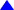## Econ 3788           Practice Problems 1             Dr. Usip Ch. 1: Conceptual Questions

Question 1. Use the information in Table 1.6 of page 22 to answer these questions.
1. What is the population of interest for this study?
2. What is the population size (N)?
3. What is the sample size (n)?
4. How many variables are associated with the dataset?
5. Which variables are (i) Quantitative and why?       (ii) Qualitative and Why?
6. Is the dataset a time-series or cross-sectional? Why?
7. What percentage of the cars are 'Compact'?
Hint: use the following coding scheme: Compact-coded = 1 if a car is Compact.
= 0 if otherwise.
(i.e. a car is Large or Midsize).
8. What percentage of the cars use 'Regular' gas?
Hint: use the following coding scheme: Regular-coded = 1 if a car uses Regular gas.
= 0 if otherwise.
(i.e. a car uses Premium or Diesel).
9. You are paid to find the average highway MPG of all cars, regardless of brand.
a. What is the parameter of interest for this study?
b. Which 'statistic' would you use for making inference about the parameter?
c. Discuss briefly the inferential process.
Hint: Highlight the key ideas of statistical inference in your discussion.

Question 2. Do problem # 13, p. 25.

Chs. 2 & 3: Applications of Descriptive Statistics

Question 1. Problem # 23, p. 54.
a. Question in the text (Hint: Use a class width of 3 and the first class from 0 - 2.9)
b. Question in the text
c. Question in the text
d. Question in the text
e. From the histogram, describe the type of skewness in the distribution data
f.  Compute the basic statistics (mean. median, mode, standard deviation, coefficient of skewness) for PC usage (in hours) during a week.
ii. Are there outliers in the data? Please justify.
Hint: Compute the standardized value or Z-score for the lowest and the highest values in the data.

Question 2.
Consider the following dataset:

 Wife's Age 22 27 25 32 34 25 Husband's Age 24 33 28 30 40 25

1. Use the mean age to explain the distribution of age among the wives vis-a-vis the husbands
2. Use the median age to explain the distribution of age among the wives vis-a-vis the husbands
3. What are the social implications of your findings in parts1 and 2 above?
4. Is the relative spread in the ages of women greater or smaller than the relative spread in the ages of men? Use the coefficient of variation to justify your answer.
Hint: Use the coefficient of variation.

Ch. 4: Probability Problems
Question.
A financial manager made two investments - one in oil industry, and one in the municipal bonds. After a one-year period, each of the investments will be classified as either successful (S) or unsuccessful (U). Consider the making of the two investments as an experiment.
1. This experiment involves how many steps?
2. How many experimental outcomes are possible for this experiment?
3. (a). Use a tree diagram to show all the possible outcomes; (b) List the outcomes in the standard format.
4. What is the probability that only one investment will be successful after one year?
5. What is the probability that none of the investments will be successful after one year?top or Back to Assignments/What is New or Home page